Скачать презентацию CMOS Analog Integrated Circuits Part 4 Operational Amplifiers

8791c8bf0d190f52514576b04f5ee1cb.ppt

• Количество слайдов: 45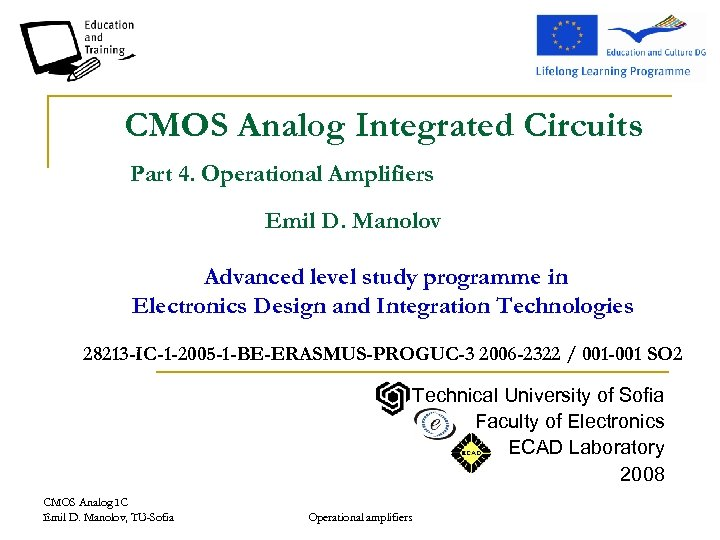CMOS Analog Integrated Circuits Part 4. Operational Amplifiers Emil D. Manolov Advanced level study programme in Electronics Design and Integration Technologies 28213 -IC-1 -2005 -1 -BE-ERASMUS-PROGUC-3 2006 -2322 / 001 -001 SO 2 Technical University of Sofia Faculty of Electronics ECAD Laboratory 2008 CMOS Analog IC Emil D. Manolov, TU-Sofia Operational amplifiers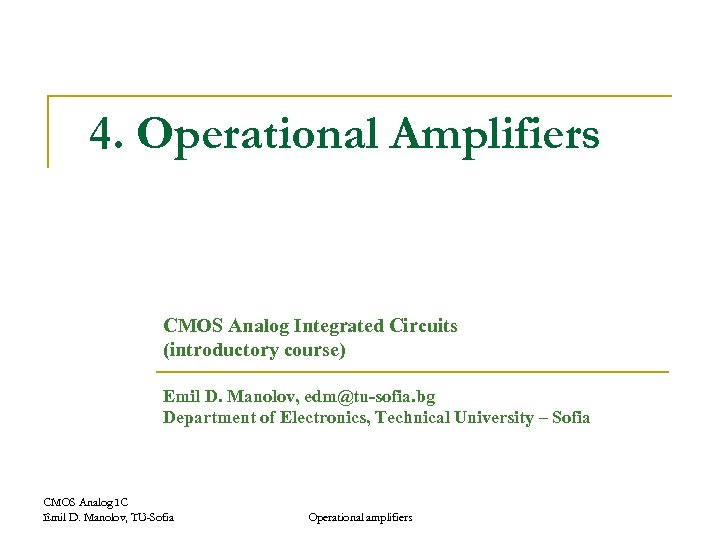4. Operational Amplifiers CMOS Analog Integrated Circuits (introductory course) Emil D. Manolov, [email protected] bg Department of Electronics, Technical University – Sofia CMOS Analog IC Emil D. Manolov, TU-Sofia Operational amplifiers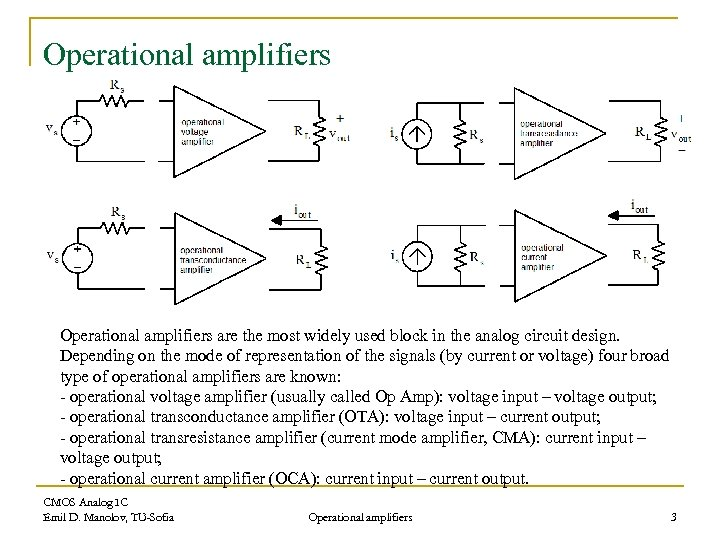Operational amplifiers are the most widely used block in the analog circuit design. Depending on the mode of representation of the signals (by current or voltage) four broad type of operational amplifiers are known: - operational voltage amplifier (usually called Op Amp): voltage input – voltage output; - operational transconductance amplifier (OTA): voltage input – current output; - operational transresistance amplifier (current mode amplifier, CMA): current input – voltage output; - operational current amplifier (OCA): current input – current output. CMOS Analog IC Emil D. Manolov, TU-Sofia Operational amplifiers 3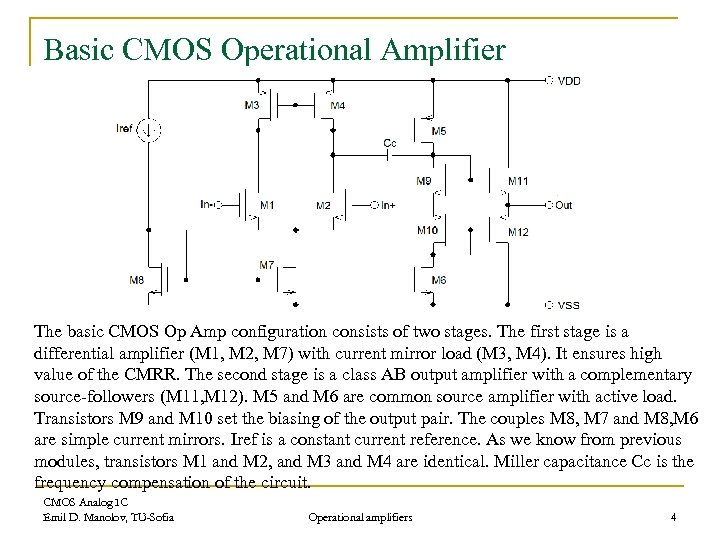Basic CMOS Operational Amplifier The basic CMOS Op Amp configuration consists of two stages. The first stage is a differential amplifier (M 1, M 2, M 7) with current mirror load (M 3, M 4). It ensures high value of the CMRR. The second stage is a class AB output amplifier with a complementary source-followers (M 11, M 12). M 5 and M 6 are common source amplifier with active load. Transistors M 9 and M 10 set the biasing of the output pair. The couples M 8, M 7 and M 8, M 6 are simple current mirrors. Iref is a constant current reference. As we know from previous modules, transistors M 1 and M 2, and M 3 and M 4 are identical. Miller capacitance Cc is the frequency compensation of the circuit. CMOS Analog IC Emil D. Manolov, TU-Sofia Operational amplifiers 4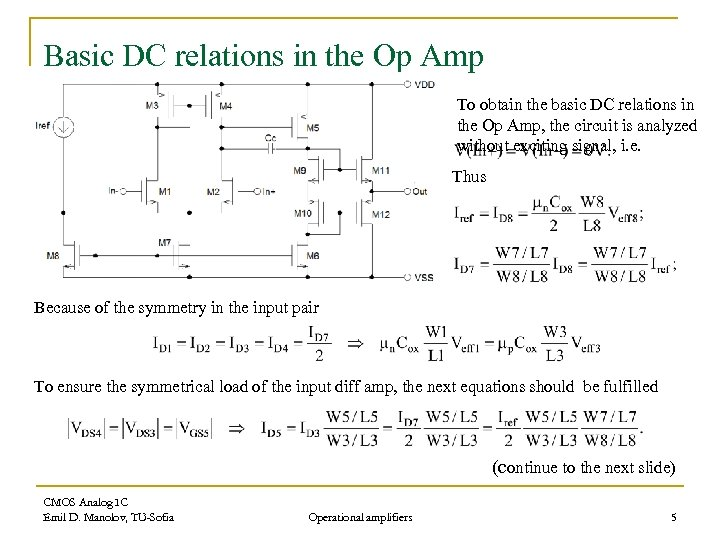Basic DC relations in the Op Amp To obtain the basic DC relations in the Op Amp, the circuit is analyzed without exciting signal, i. e. Thus Because of the symmetry in the input pair To ensure the symmetrical load of the input diff amp, the next equations should be fulfilled (continue to the next slide) CMOS Analog IC Emil D. Manolov, TU-Sofia Operational amplifiers 5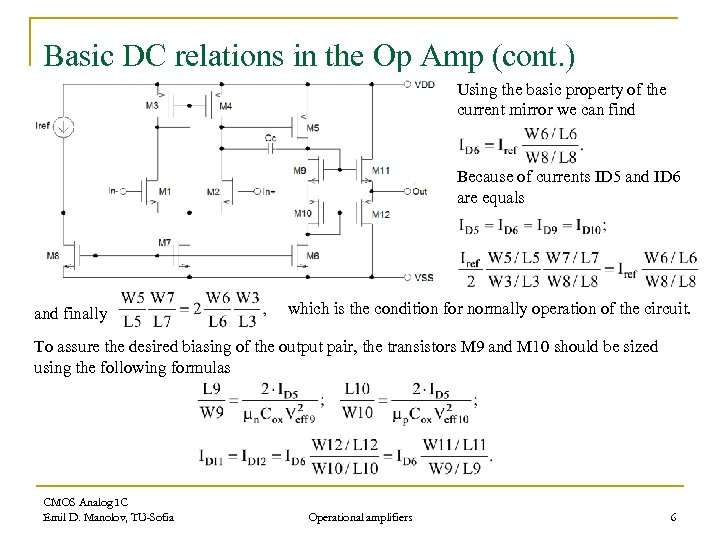Basic DC relations in the Op Amp (cont. ) Using the basic property of the current mirror we can find Because of currents ID 5 and ID 6 are equals and finally which is the condition for normally operation of the circuit. To assure the desired biasing of the output pair, the transistors M 9 and M 10 should be sized using the following formulas CMOS Analog IC Emil D. Manolov, TU-Sofia Operational amplifiers 6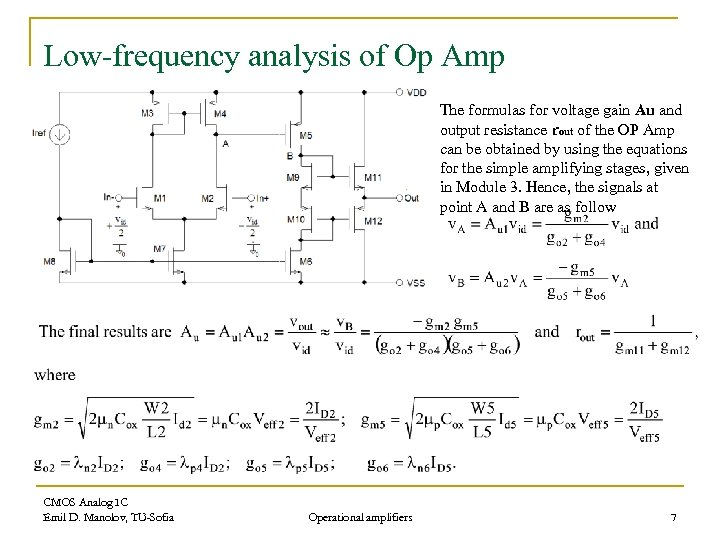Low-frequency analysis of Op Amp The formulas for voltage gain Au and output resistance rout of the OP Amp can be obtained by using the equations for the simple amplifying stages, given in Module 3. Hence, the signals at point A and B are as follow CMOS Analog IC Emil D. Manolov, TU-Sofia Operational amplifiers 7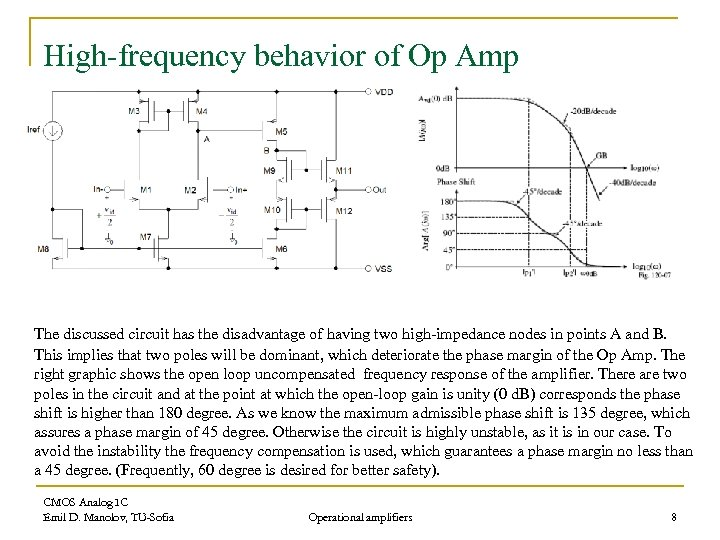High-frequency behavior of Op Amp The discussed circuit has the disadvantage of having two high-impedance nodes in points A and B. This implies that two poles will be dominant, which deteriorate the phase margin of the Op Amp. The right graphic shows the open loop uncompensated frequency response of the amplifier. There are two poles in the circuit and at the point at which the open-loop gain is unity (0 d. B) corresponds the phase shift is higher than 180 degree. As we know the maximum admissible phase shift is 135 degree, which assures a phase margin of 45 degree. Otherwise the circuit is highly unstable, as it is in our case. To avoid the instability the frequency compensation is used, which guarantees a phase margin no less than a 45 degree. (Frequently, 60 degree is desired for better safety). CMOS Analog IC Emil D. Manolov, TU-Sofia Operational amplifiers 8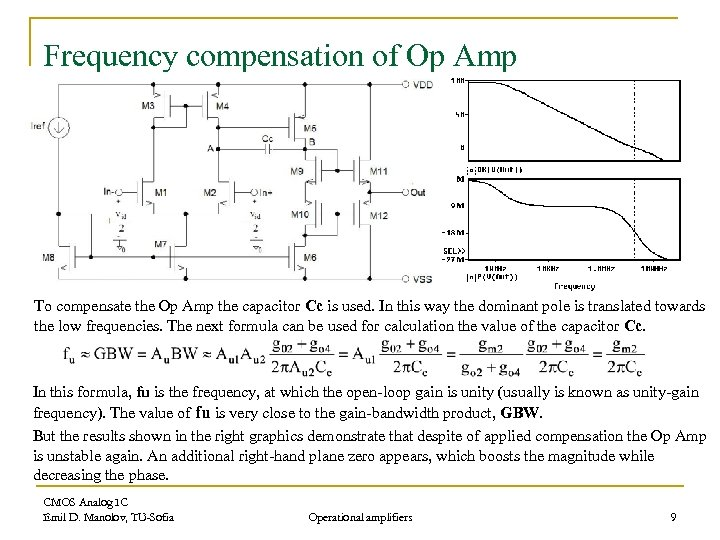Frequency compensation of Op Amp To compensate the Op Amp the capacitor Cc is used. In this way the dominant pole is translated towards the low frequencies. The next formula can be used for calculation the value of the capacitor Cc. In this formula, fu is the frequency, at which the open-loop gain is unity (usually is known as unity-gain frequency). The value of fu is very close to the gain-bandwidth product, GBW. But the results shown in the right graphics demonstrate that despite of applied compensation the Op Amp is unstable again. An additional right-hand plane zero appears, which boosts the magnitude while decreasing the phase. CMOS Analog IC Emil D. Manolov, TU-Sofia Operational amplifiers 9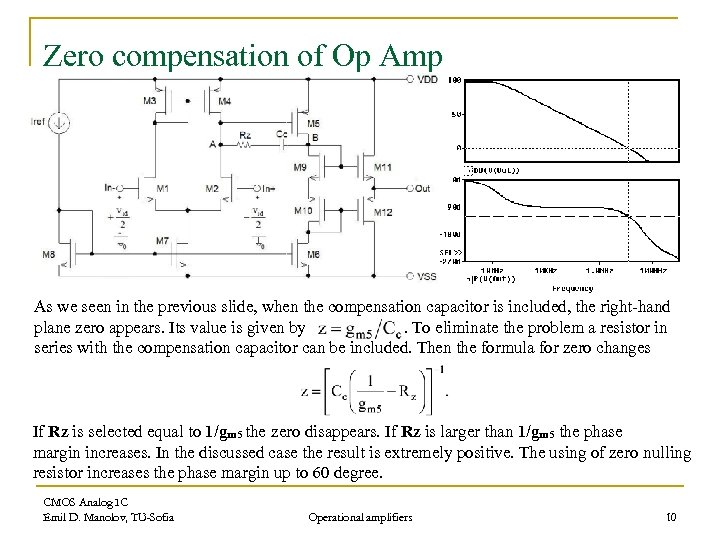Zero compensation of Op Amp As we seen in the previous slide, when the compensation capacitor is included, the right-hand plane zero appears. Its value is given by. To eliminate the problem a resistor in series with the compensation capacitor can be included. Then the formula for zero changes If Rz is selected equal to 1/gm 5 the zero disappears. If Rz is larger than 1/gm 5 the phase margin increases. In the discussed case the result is extremely positive. The using of zero nulling resistor increases the phase margin up to 60 degree. CMOS Analog IC Emil D. Manolov, TU-Sofia Operational amplifiers 10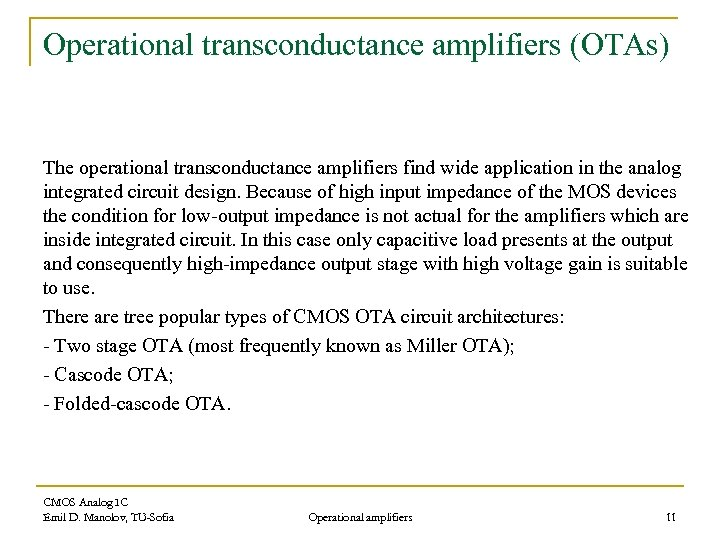Operational transconductance amplifiers (OTAs) The operational transconductance amplifiers find wide application in the analog integrated circuit design. Because of high input impedance of the MOS devices the condition for low-output impedance is not actual for the amplifiers which are inside integrated circuit. In this case only capacitive load presents at the output and consequently high-impedance output stage with high voltage gain is suitable to use. There are tree popular types of CMOS OTA circuit architectures: - Two stage OTA (most frequently known as Miller OTA); - Cascode OTA; - Folded-cascode OTA. CMOS Analog IC Emil D. Manolov, TU-Sofia Operational amplifiers 11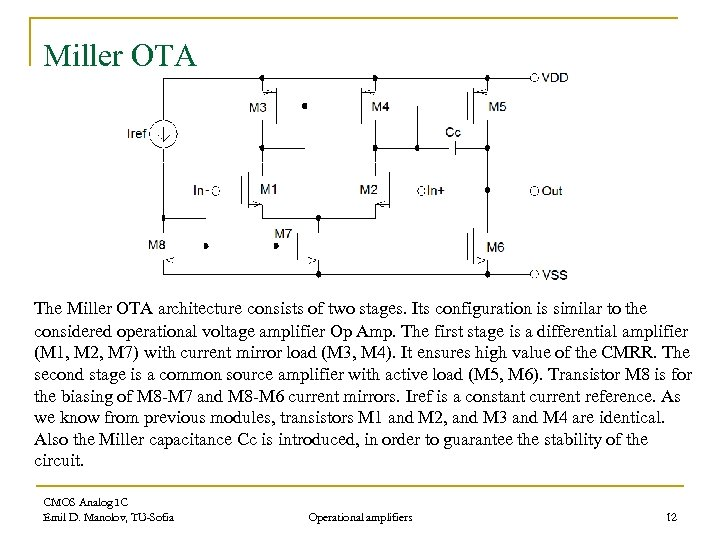Miller OTA The Miller OTA architecture consists of two stages. Its configuration is similar to the considered operational voltage amplifier Op Amp. The first stage is a differential amplifier (M 1, M 2, M 7) with current mirror load (M 3, M 4). It ensures high value of the CMRR. The second stage is a common source amplifier with active load (M 5, M 6). Transistor M 8 is for the biasing of M 8 -M 7 and M 8 -M 6 current mirrors. Iref is a constant current reference. As we know from previous modules, transistors M 1 and M 2, and M 3 and M 4 are identical. Also the Miller capacitance Cc is introduced, in order to guarantee the stability of the circuit. CMOS Analog IC Emil D. Manolov, TU-Sofia Operational amplifiers 12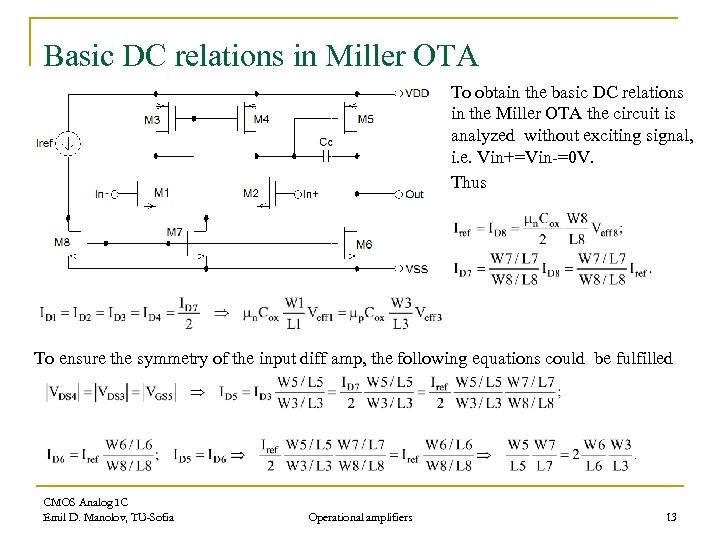Basic DC relations in Miller OTA To obtain the basic DC relations in the Miller OTA the circuit is analyzed without exciting signal, i. e. Vin+=Vin-=0 V. Thus To ensure the symmetry of the input diff amp, the following equations could be fulfilled CMOS Analog IC Emil D. Manolov, TU-Sofia Operational amplifiers 13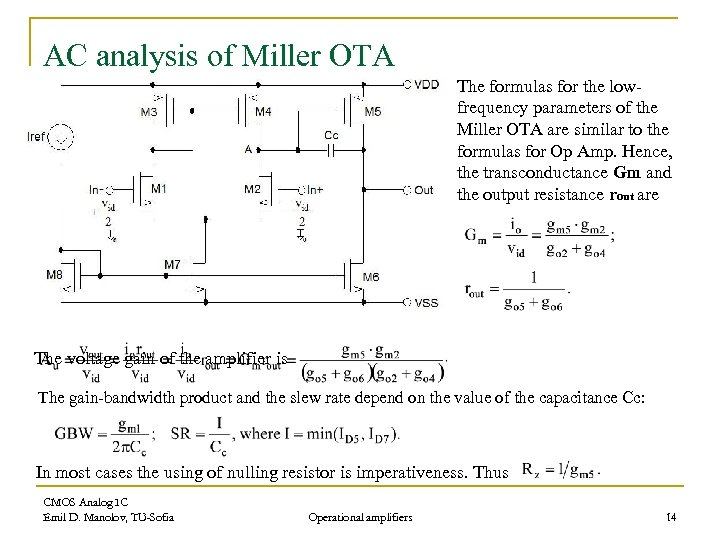AC analysis of Miller OTA The formulas for the lowfrequency parameters of the Miller OTA are similar to the formulas for Op Amp. Hence, the transconductance Gm and the output resistance rout are The voltage gain of the amplifier is The gain-bandwidth product and the slew rate depend on the value of the capacitance Cc: In most cases the using of nulling resistor is imperativeness. Thus CMOS Analog IC Emil D. Manolov, TU-Sofia Operational amplifiers 14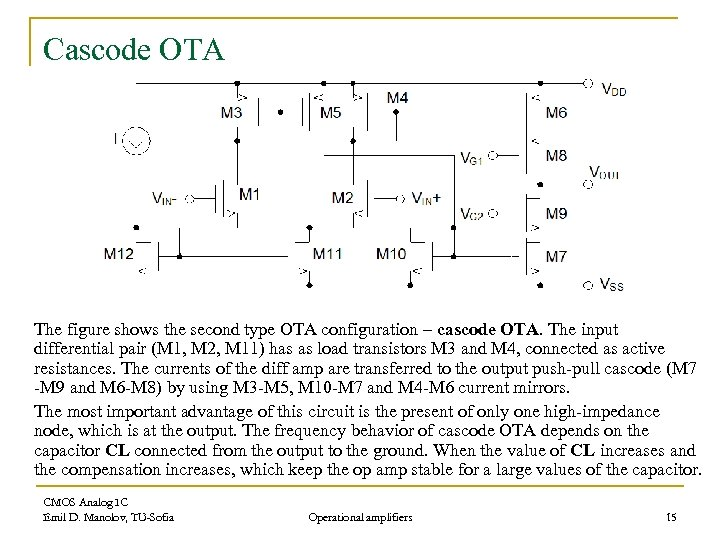Cascode OTA The figure shows the second type OTA configuration – cascode OTA. The input differential pair (M 1, M 2, M 11) has as load transistors M 3 and M 4, connected as active resistances. The currents of the diff amp are transferred to the output push-pull cascode (M 7 -M 9 and M 6 -M 8) by using M 3 -M 5, M 10 -M 7 and M 4 -M 6 current mirrors. The most important advantage of this circuit is the present of only one high-impedance node, which is at the output. The frequency behavior of cascode OTA depends on the capacitor CL connected from the output to the ground. When the value of CL increases and the compensation increases, which keep the op amp stable for a large values of the capacitor. CMOS Analog IC Emil D. Manolov, TU-Sofia Operational amplifiers 15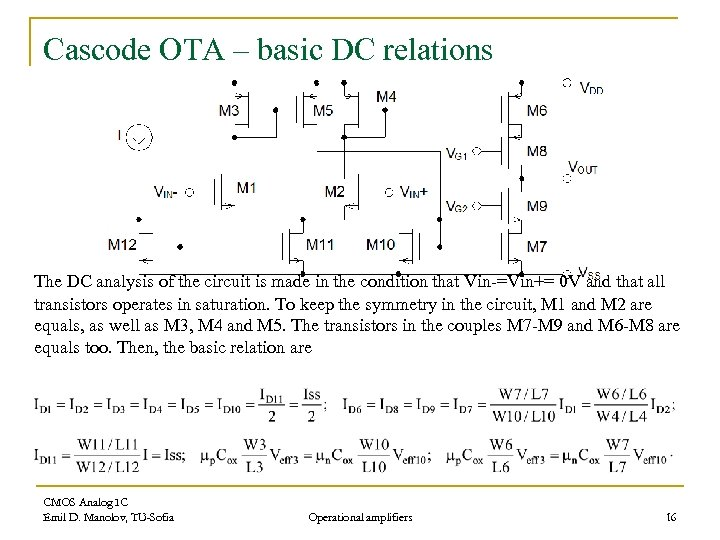Cascode OTA – basic DC relations The DC analysis of the circuit is made in the condition that Vin-=Vin+= 0 V and that all transistors operates in saturation. To keep the symmetry in the circuit, M 1 and M 2 are equals, as well as M 3, M 4 and M 5. The transistors in the couples M 7 -M 9 and M 6 -M 8 are equals too. Then, the basic relation are CMOS Analog IC Emil D. Manolov, TU-Sofia Operational amplifiers 16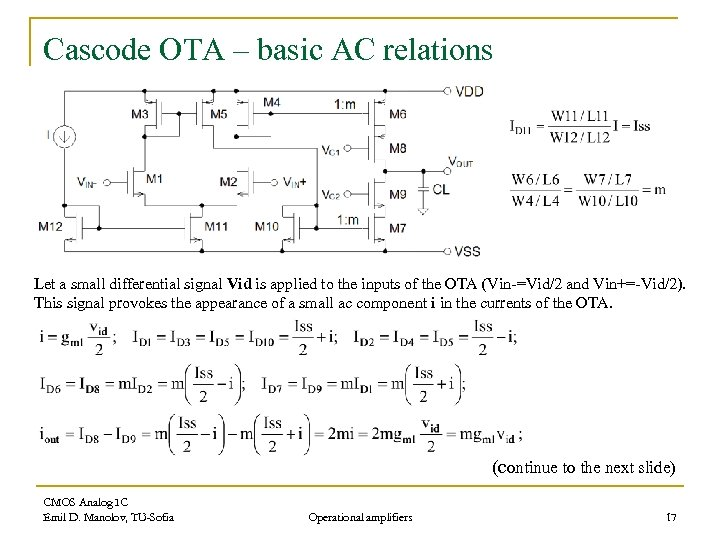Cascode OTA – basic AC relations Let a small differential signal Vid is applied to the inputs of the OTA (Vin-=Vid/2 and Vin+=-Vid/2). This signal provokes the appearance of a small ac component i in the currents of the OTA. (continue to the next slide) CMOS Analog IC Emil D. Manolov, TU-Sofia Operational amplifiers 17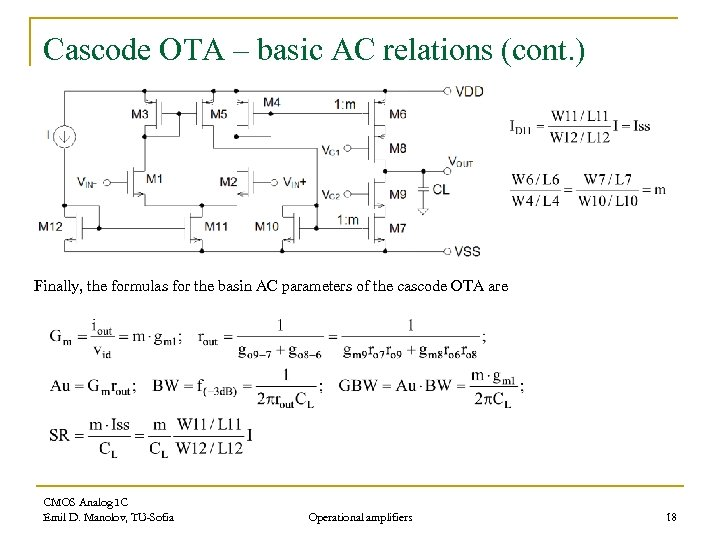Cascode OTA – basic AC relations (cont. ) Finally, the formulas for the basin AC parameters of the cascode OTA are CMOS Analog IC Emil D. Manolov, TU-Sofia Operational amplifiers 18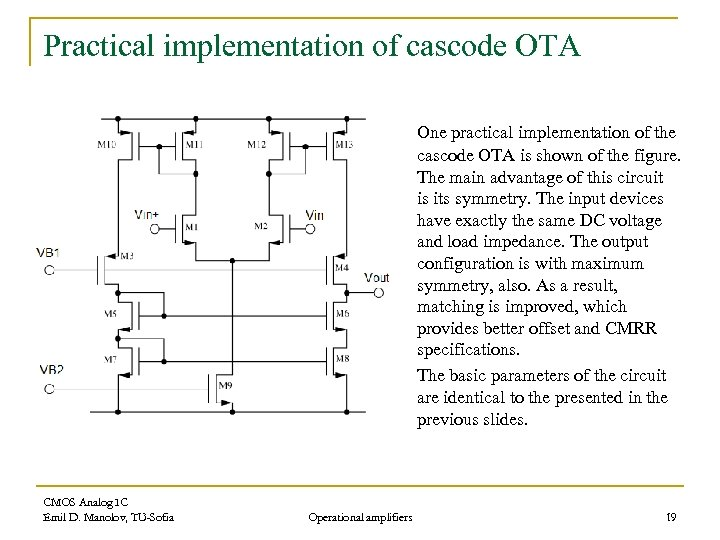Practical implementation of cascode OTA One practical implementation of the cascode OTA is shown of the figure. The main advantage of this circuit is its symmetry. The input devices have exactly the same DC voltage and load impedance. The output configuration is with maximum symmetry, also. As a result, matching is improved, which provides better offset and CMRR specifications. The basic parameters of the circuit are identical to the presented in the previous slides. CMOS Analog IC Emil D. Manolov, TU-Sofia Operational amplifiers 19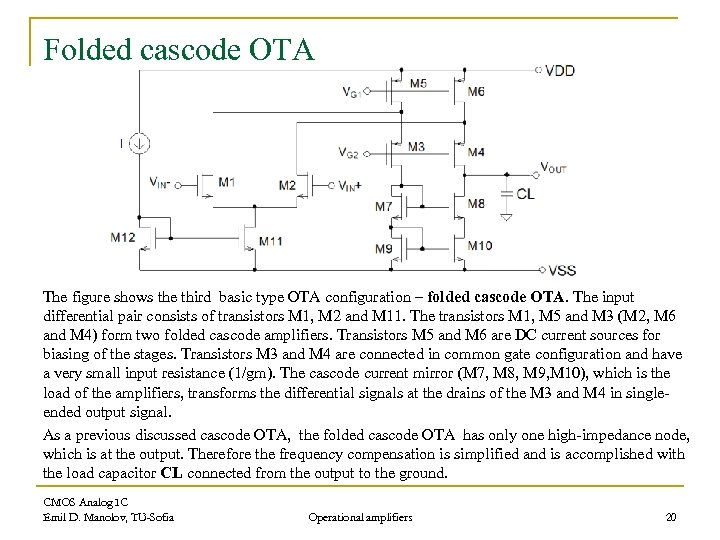Folded cascode OTA The figure shows the third basic type OTA configuration – folded cascode OTA. The input differential pair consists of transistors M 1, M 2 and M 11. The transistors M 1, M 5 and M 3 (M 2, M 6 and M 4) form two folded cascode amplifiers. Transistors M 5 and M 6 are DC current sources for biasing of the stages. Transistors M 3 and M 4 are connected in common gate configuration and have a very small input resistance (1/gm). The cascode current mirror (M 7, M 8, M 9, M 10), which is the load of the amplifiers, transforms the differential signals at the drains of the M 3 and M 4 in singleended output signal. As a previous discussed cascode OTA, the folded cascode OTA has only one high-impedance node, which is at the output. Therefore the frequency compensation is simplified and is accomplished with the load capacitor CL connected from the output to the ground. CMOS Analog IC Emil D. Manolov, TU-Sofia Operational amplifiers 20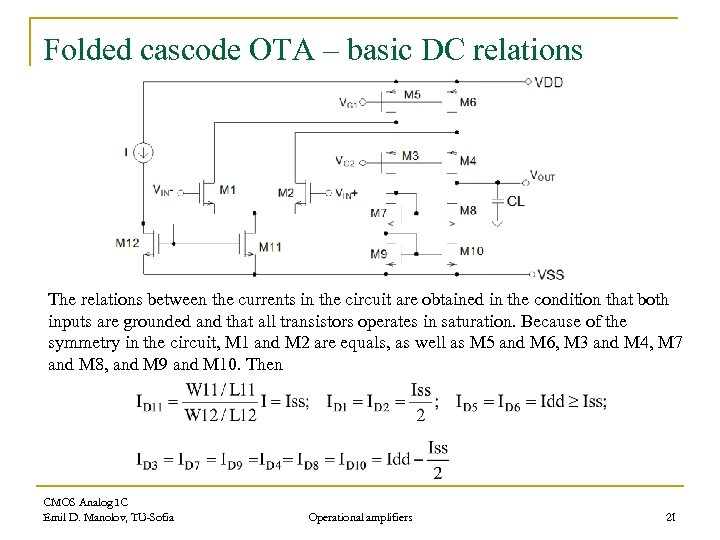Folded cascode OTA – basic DC relations The relations between the currents in the circuit are obtained in the condition that both inputs are grounded and that all transistors operates in saturation. Because of the symmetry in the circuit, M 1 and M 2 are equals, as well as M 5 and M 6, M 3 and M 4, M 7 and M 8, and M 9 and M 10. Then CMOS Analog IC Emil D. Manolov, TU-Sofia Operational amplifiers 21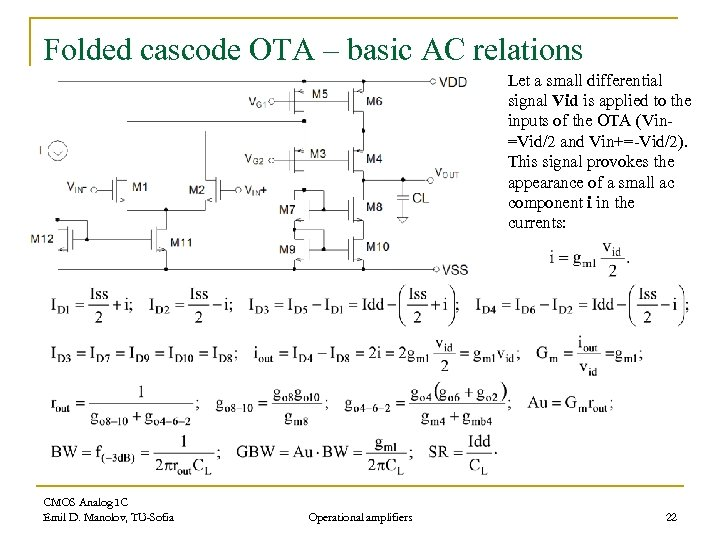Folded cascode OTA – basic AC relations Let a small differential signal Vid is applied to the inputs of the OTA (Vin=Vid/2 and Vin+=-Vid/2). This signal provokes the appearance of a small ac component i in the currents: CMOS Analog IC Emil D. Manolov, TU-Sofia Operational amplifiers 22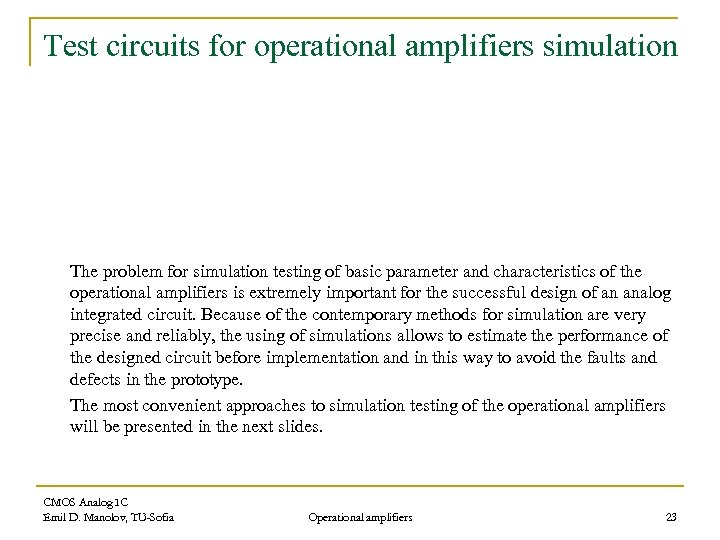Test circuits for operational amplifiers simulation The problem for simulation testing of basic parameter and characteristics of the operational amplifiers is extremely important for the successful design of an analog integrated circuit. Because of the contemporary methods for simulation are very precise and reliably, the using of simulations allows to estimate the performance of the designed circuit before implementation and in this way to avoid the faults and defects in the prototype. The most convenient approaches to simulation testing of the operational amplifiers will be presented in the next slides. CMOS Analog IC Emil D. Manolov, TU-Sofia Operational amplifiers 23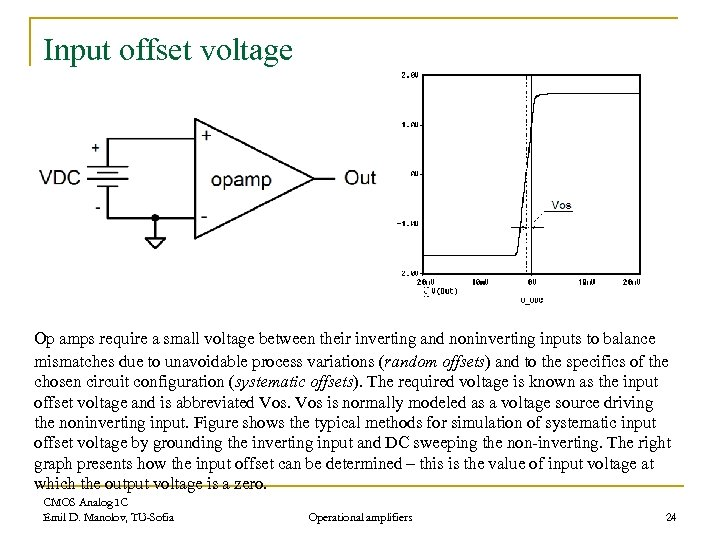Input offset voltage Op amps require a small voltage between their inverting and noninverting inputs to balance mismatches due to unavoidable process variations (random offsets) and to the specifics of the chosen circuit configuration (systematic offsets). The required voltage is known as the input offset voltage and is abbreviated Vos is normally modeled as a voltage source driving the noninverting input. Figure shows the typical methods for simulation of systematic input offset voltage by grounding the inverting input and DC sweeping the non-inverting. The right graph presents how the input offset can be determined – this is the value of input voltage at which the output voltage is a zero. CMOS Analog IC Emil D. Manolov, TU-Sofia Operational amplifiers 24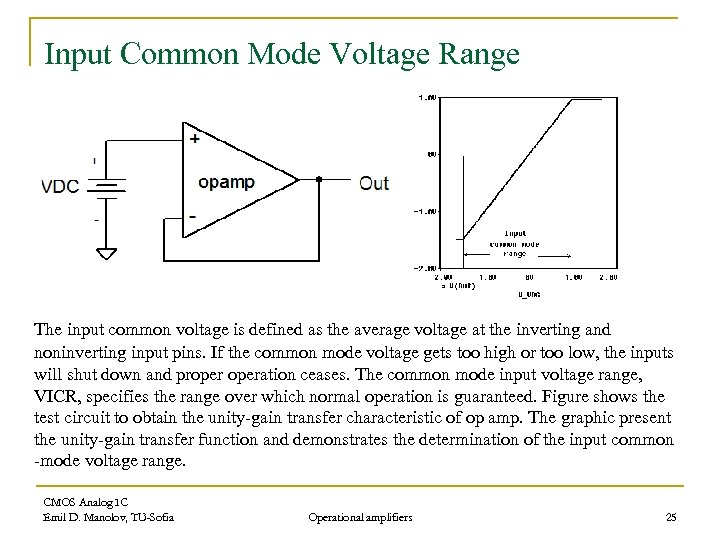Input Common Mode Voltage Range The input common voltage is defined as the average voltage at the inverting and noninverting input pins. If the common mode voltage gets too high or too low, the inputs will shut down and properation ceases. The common mode input voltage range, VICR, specifies the range over which normal operation is guaranteed. Figure shows the test circuit to obtain the unity-gain transfer characteristic of op amp. The graphic present the unity-gain transfer function and demonstrates the determination of the input common -mode voltage range. CMOS Analog IC Emil D. Manolov, TU-Sofia Operational amplifiers 25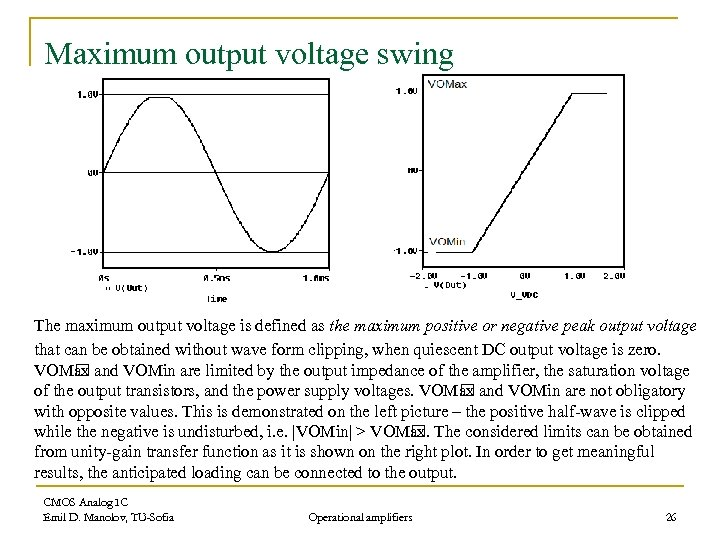Maximum output voltage swing The maximum output voltage is defined as the maximum positive or negative peak output voltage that can be obtained without wave form clipping, when quiescent DC output voltage is zero. VOM and VOMin are limited by the output impedance of the amplifier, the saturation voltage ax of the output transistors, and the power supply voltages. VOM and VOMin are not obligatory ax with opposite values. This is demonstrated on the left picture – the positive half-wave is clipped while the negative is undisturbed, i. e. |VOMin| > VOM The considered limits can be obtained ax. from unity-gain transfer function as it is shown on the right plot. In order to get meaningful results, the anticipated loading can be connected to the output. CMOS Analog IC Emil D. Manolov, TU-Sofia Operational amplifiers 26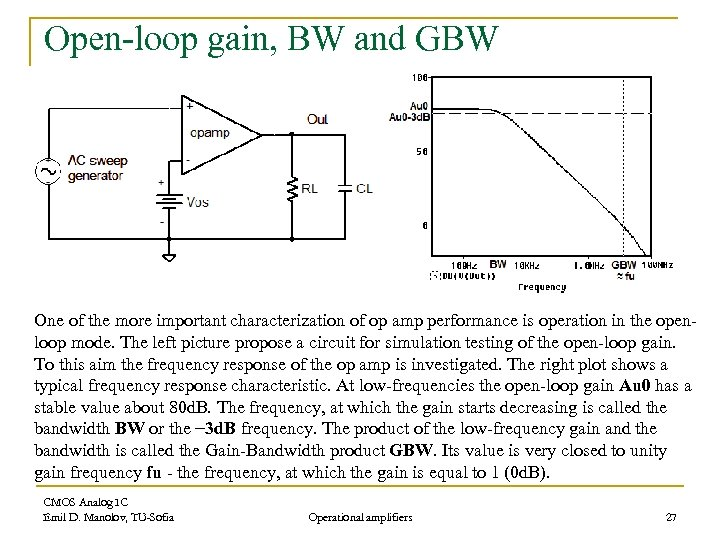Open-loop gain, BW and GBW One of the more important characterization of op amp performance is operation in the openloop mode. The left picture propose a circuit for simulation testing of the open-loop gain. To this aim the frequency response of the op amp is investigated. The right plot shows a typical frequency response characteristic. At low-frequencies the open-loop gain Au 0 has a stable value about 80 d. B. The frequency, at which the gain starts decreasing is called the bandwidth BW or the − 3 d. B frequency. The product of the low-frequency gain and the bandwidth is called the Gain-Bandwidth product GBW. Its value is very closed to unity gain frequency fu - the frequency, at which the gain is equal to 1 (0 d. B). CMOS Analog IC Emil D. Manolov, TU-Sofia Operational amplifiers 27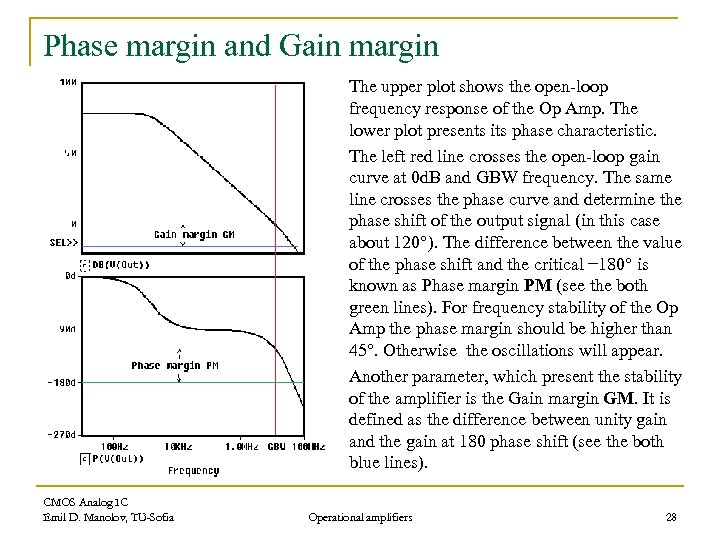Phase margin and Gain margin The upper plot shows the open-loop frequency response of the Op Amp. The lower plot presents its phase characteristic. The left red line crosses the open-loop gain curve at 0 d. B and GBW frequency. The same line crosses the phase curve and determine the phase shift of the output signal (in this case about 120°). The difference between the value of the phase shift and the critical − 180° is known as Phase margin PM (see the both green lines). For frequency stability of the Op Amp the phase margin should be higher than 45°. Otherwise the oscillations will appear. Another parameter, which present the stability of the amplifier is the Gain margin GM. It is defined as the difference between unity gain and the gain at 180 phase shift (see the both blue lines). CMOS Analog IC Emil D. Manolov, TU-Sofia Operational amplifiers 28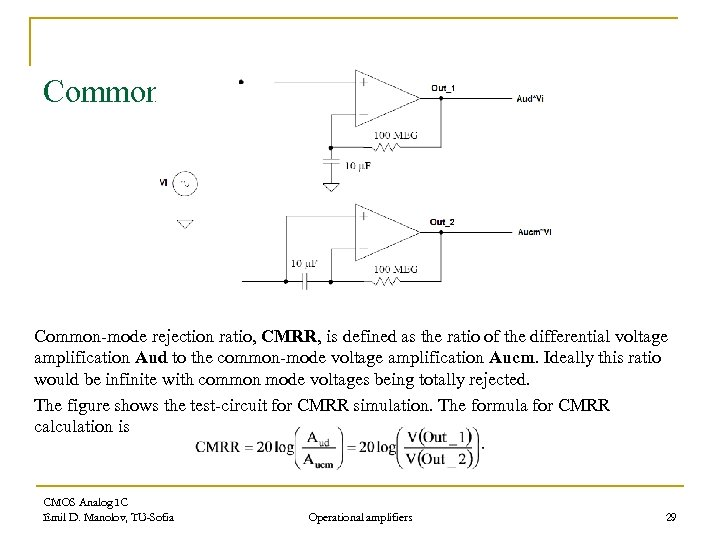Common mode rejection ratio Common-mode rejection ratio, CMRR, is defined as the ratio of the differential voltage amplification Aud to the common-mode voltage amplification Aucm. Ideally this ratio would be infinite with common mode voltages being totally rejected. The figure shows the test-circuit for CMRR simulation. The formula for CMRR calculation is CMOS Analog IC Emil D. Manolov, TU-Sofia Operational amplifiers 29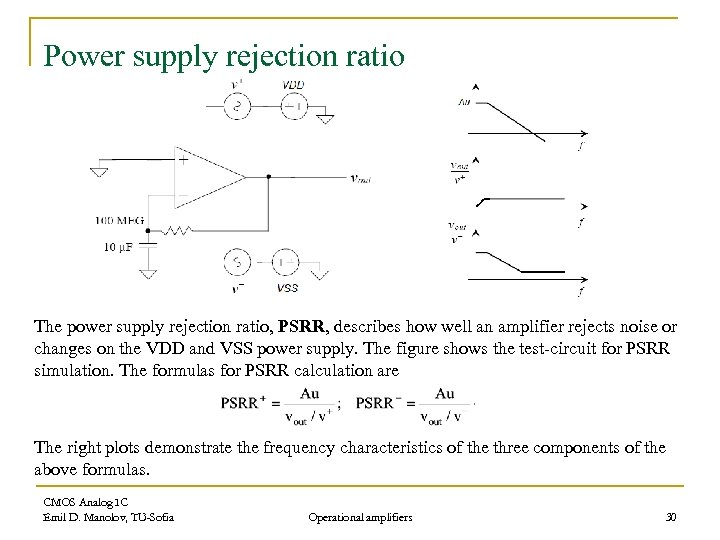Power supply rejection ratio The power supply rejection ratio, PSRR, describes how well an amplifier rejects noise or changes on the VDD and VSS power supply. The figure shows the test-circuit for PSRR simulation. The formulas for PSRR calculation are The right plots demonstrate the frequency characteristics of the three components of the above formulas. CMOS Analog IC Emil D. Manolov, TU-Sofia Operational amplifiers 30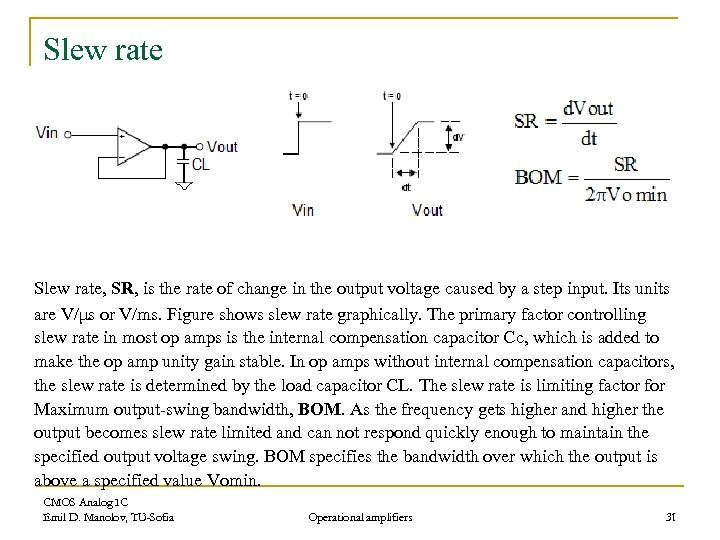Slew rate, SR, is the rate of change in the output voltage caused by a step input. Its units are V/μs or V/ms. Figure shows slew rate graphically. The primary factor controlling slew rate in most op amps is the internal compensation capacitor Cc, which is added to make the op amp unity gain stable. In op amps without internal compensation capacitors, the slew rate is determined by the load capacitor CL. The slew rate is limiting factor for Maximum output-swing bandwidth, BOM. As the frequency gets higher and higher the output becomes slew rate limited and can not respond quickly enough to maintain the specified output voltage swing. BOM specifies the bandwidth over which the output is above a specified value Vomin. CMOS Analog IC Emil D. Manolov, TU-Sofia Operational amplifiers 31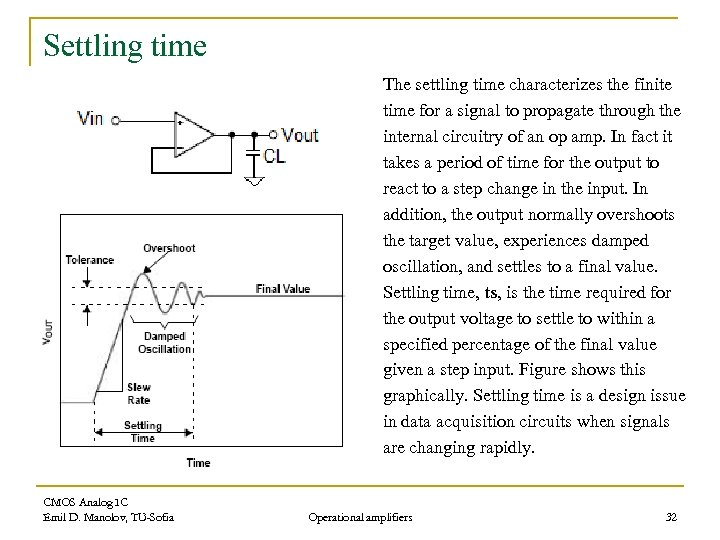Settling time The settling time characterizes the finite time for a signal to propagate through the internal circuitry of an op amp. In fact it takes a period of time for the output to react to a step change in the input. In addition, the output normally overshoots the target value, experiences damped oscillation, and settles to a final value. Settling time, ts, is the time required for the output voltage to settle to within a specified percentage of the final value given a step input. Figure shows this graphically. Settling time is a design issue in data acquisition circuits when signals are changing rapidly. CMOS Analog IC Emil D. Manolov, TU-Sofia Operational amplifiers 32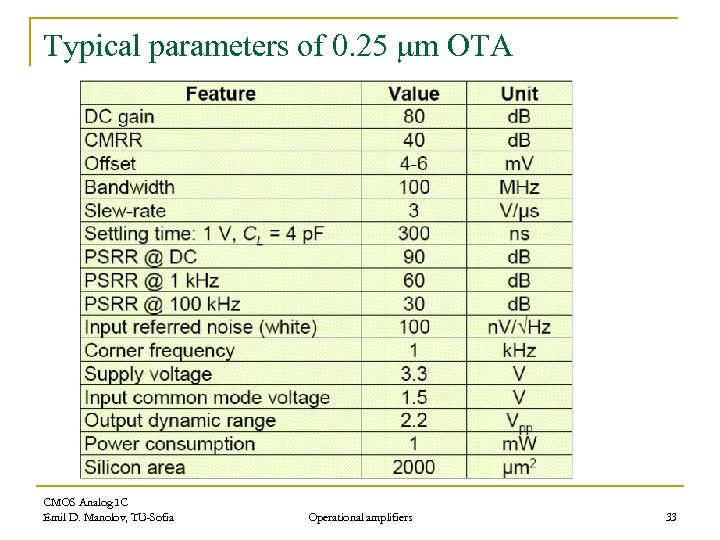Typical parameters of 0. 25 mm OTA CMOS Analog IC Emil D. Manolov, TU-Sofia Operational amplifiers 33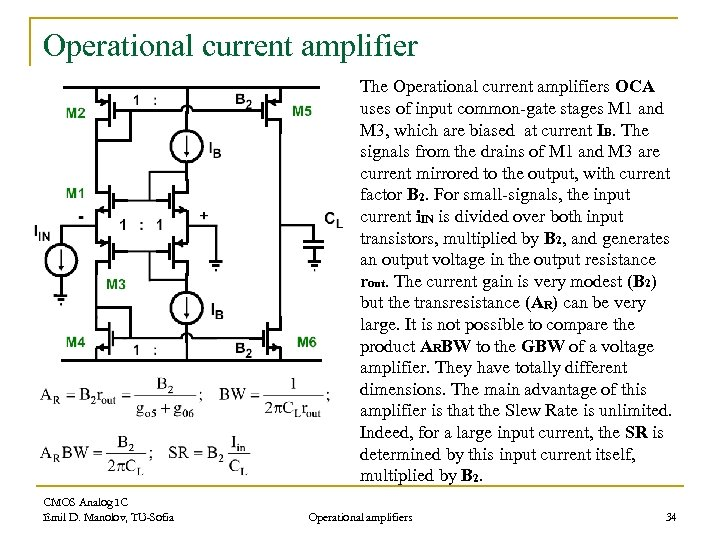Operational current amplifier The Operational current amplifiers OCA uses of input common-gate stages M 1 and M 3, which are biased at current IB. The signals from the drains of M 1 and M 3 are current mirrored to the output, with current factor B 2. For small-signals, the input current i. IN is divided over both input transistors, multiplied by B 2, and generates an output voltage in the output resistance rout. The current gain is very modest (B 2) but the transresistance (AR) can be very large. It is not possible to compare the product ARBW to the GBW of a voltage amplifier. They have totally different dimensions. The main advantage of this amplifier is that the Slew Rate is unlimited. Indeed, for a large input current, the SR is determined by this input current itself, multiplied by B 2. CMOS Analog IC Emil D. Manolov, TU-Sofia Operational amplifiers 34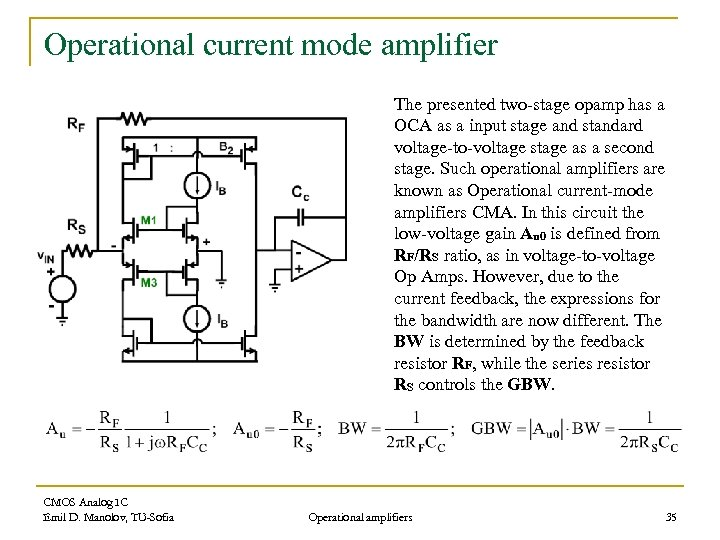Operational current mode amplifier The presented two-stage opamp has a OCA as a input stage and standard voltage-to-voltage stage as a second stage. Such operational amplifiers are known as Operational current-mode amplifiers CMA. In this circuit the low-voltage gain Au 0 is defined from RF/RS ratio, as in voltage-to-voltage Op Amps. However, due to the current feedback, the expressions for the bandwidth are now different. The BW is determined by the feedback resistor RF, while the series resistor RS controls the GBW. CMOS Analog IC Emil D. Manolov, TU-Sofia Operational amplifiers 35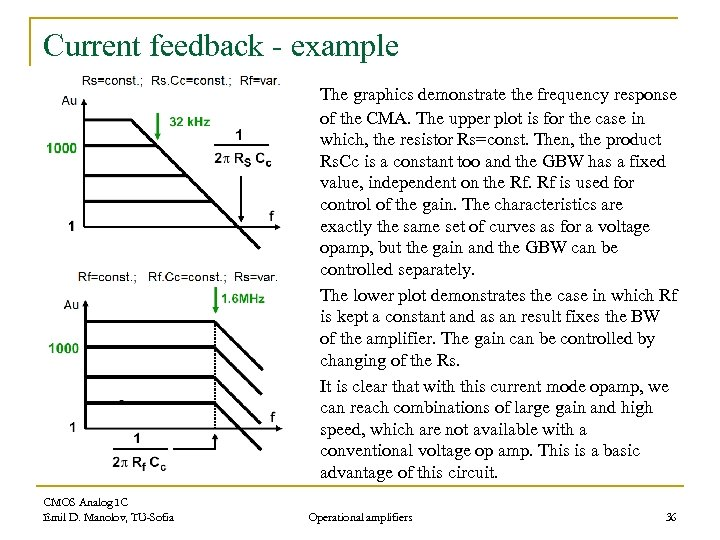Current feedback - example The graphics demonstrate the frequency response of the CMA. The upper plot is for the case in which, the resistor Rs=const. Then, the product Rs. Cc is a constant too and the GBW has a fixed value, independent on the Rf. Rf is used for control of the gain. The characteristics are exactly the same set of curves as for a voltage opamp, but the gain and the GBW can be controlled separately. The lower plot demonstrates the case in which Rf is kept a constant and as an result fixes the BW of the amplifier. The gain can be controlled by changing of the Rs. It is clear that with this current mode opamp, we can reach combinations of large gain and high speed, which are not available with a conventional voltage op amp. This is a basic advantage of this circuit. CMOS Analog IC Emil D. Manolov, TU-Sofia Operational amplifiers 36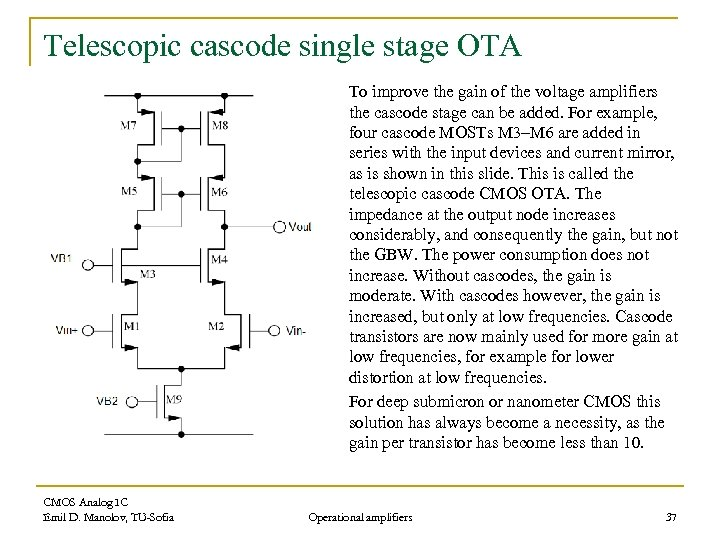Telescopic cascode single stage OTA To improve the gain of the voltage amplifiers the cascode stage can be added. For example, four cascode MOSTs M 3–M 6 are added in series with the input devices and current mirror, as is shown in this slide. This is called the telescopic cascode CMOS OTA. The impedance at the output node increases considerably, and consequently the gain, but not the GBW. The power consumption does not increase. Without cascodes, the gain is moderate. With cascodes however, the gain is increased, but only at low frequencies. Cascode transistors are now mainly used for more gain at low frequencies, for example for lower distortion at low frequencies. For deep submicron or nanometer CMOS this solution has always become a necessity, as the gain per transistor has become less than 10. CMOS Analog IC Emil D. Manolov, TU-Sofia Operational amplifiers 37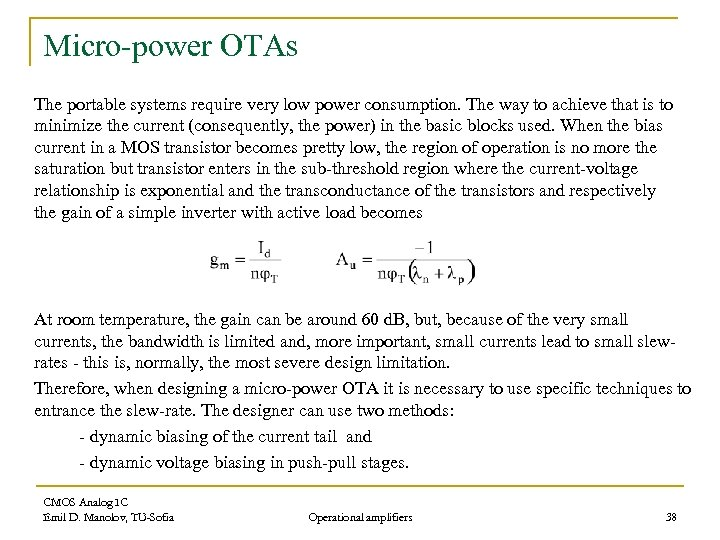Micro-power OTAs The portable systems require very low power consumption. The way to achieve that is to minimize the current (consequently, the power) in the basic blocks used. When the bias current in a MOS transistor becomes pretty low, the region of operation is no more the saturation but transistor enters in the sub-threshold region where the current-voltage relationship is exponential and the transconductance of the transistors and respectively the gain of a simple inverter with active load becomes At room temperature, the gain can be around 60 d. B, but, because of the very small currents, the bandwidth is limited and, more important, small currents lead to small slewrates - this is, normally, the most severe design limitation. Therefore, when designing a micro-power OTA it is necessary to use specific techniques to entrance the slew-rate. The designer can use two methods: - dynamic biasing of the current tail and - dynamic voltage biasing in push-pull stages. CMOS Analog IC Emil D. Manolov, TU-Sofia Operational amplifiers 38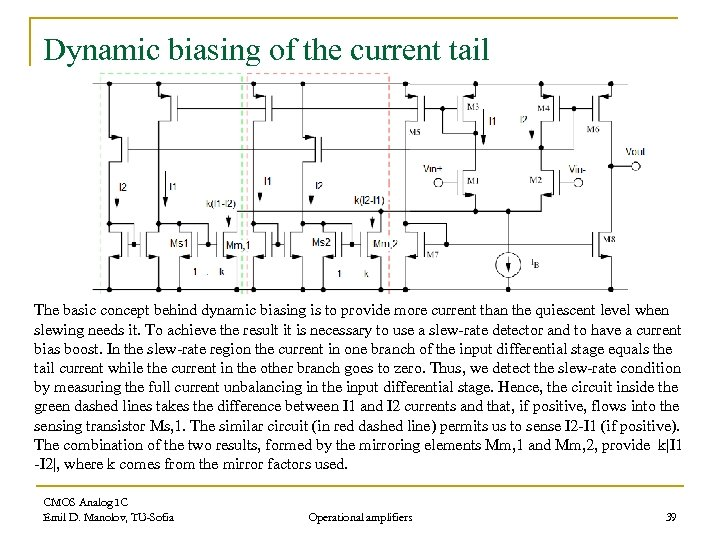Dynamic biasing of the current tail The basic concept behind dynamic biasing is to provide more current than the quiescent level when slewing needs it. To achieve the result it is necessary to use a slew-rate detector and to have a current bias boost. In the slew-rate region the current in one branch of the input differential stage equals the tail current while the current in the other branch goes to zero. Thus, we detect the slew-rate condition by measuring the full current unbalancing in the input differential stage. Hence, the circuit inside the green dashed lines takes the difference between I 1 and I 2 currents and that, if positive, flows into the sensing transistor Ms, 1. The similar circuit (in red dashed line) permits us to sense I 2 -I 1 (if positive). The combination of the two results, formed by the mirroring elements Mm, 1 and Mm, 2, provide k|I 1 -I 2|, where k comes from the mirror factors used. CMOS Analog IC Emil D. Manolov, TU-Sofia Operational amplifiers 39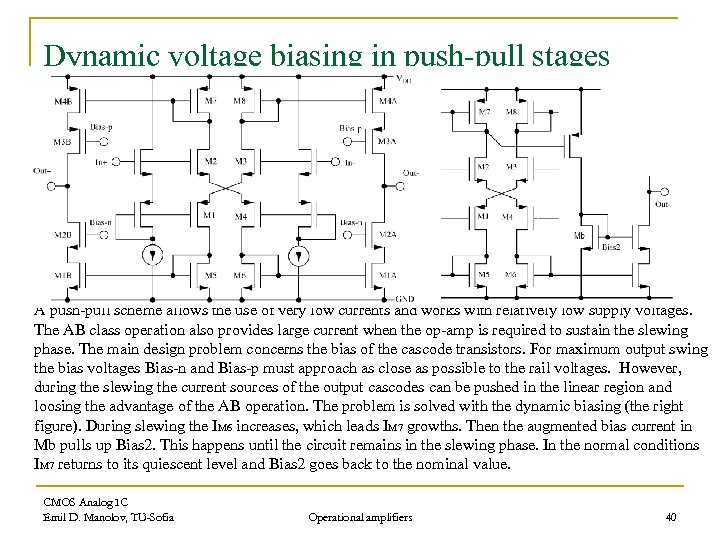Dynamic voltage biasing in push-pull stages A push-pull scheme allows the use of very low currents and works with relatively low supply voltages. The AB class operation also provides large current when the op-amp is required to sustain the slewing phase. The main design problem concerns the bias of the cascode transistors. For maximum output swing the bias voltages Bias-n and Bias-p must approach as close as possible to the rail voltages. However, during the slewing the current sources of the output cascodes can be pushed in the linear region and loosing the advantage of the AB operation. The problem is solved with the dynamic biasing (the right figure). During slewing the IM 6 increases, which leads IM 7 growths. Then the augmented bias current in Mb pulls up Bias 2. This happens until the circuit remains in the slewing phase. In the normal conditions IM 7 returns to its quiescent level and Bias 2 goes back to the nominal value. CMOS Analog IC Emil D. Manolov, TU-Sofia Operational amplifiers 40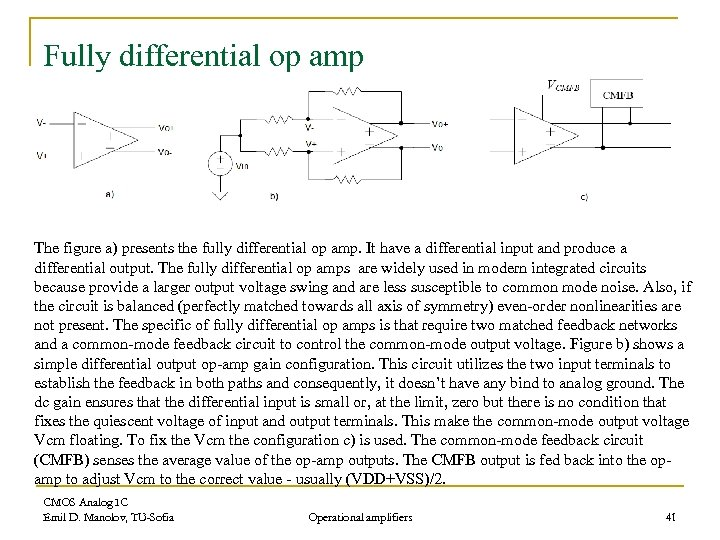Fully differential op amp The figure a) presents the fully differential op amp. It have a differential input and produce a differential output. The fully differential op amps are widely used in modern integrated circuits because provide a larger output voltage swing and are less susceptible to common mode noise. Also, if the circuit is balanced (perfectly matched towards all axis of symmetry) even-order nonlinearities are not present. The specific of fully differential op amps is that require two matched feedback networks and a common-mode feedback circuit to control the common-mode output voltage. Figure b) shows a simple differential output op-amp gain configuration. This circuit utilizes the two input terminals to establish the feedback in both paths and consequently, it doesn’t have any bind to analog ground. The dc gain ensures that the differential input is small or, at the limit, zero but there is no condition that fixes the quiescent voltage of input and output terminals. This make the common-mode output voltage Vcm floating. To fix the Vcm the configuration c) is used. The common-mode feedback circuit (CMFB) senses the average value of the op-amp outputs. The CMFB output is fed back into the opamp to adjust Vcm to the correct value - usually (VDD+VSS)/2. CMOS Analog IC Emil D. Manolov, TU-Sofia Operational amplifiers 41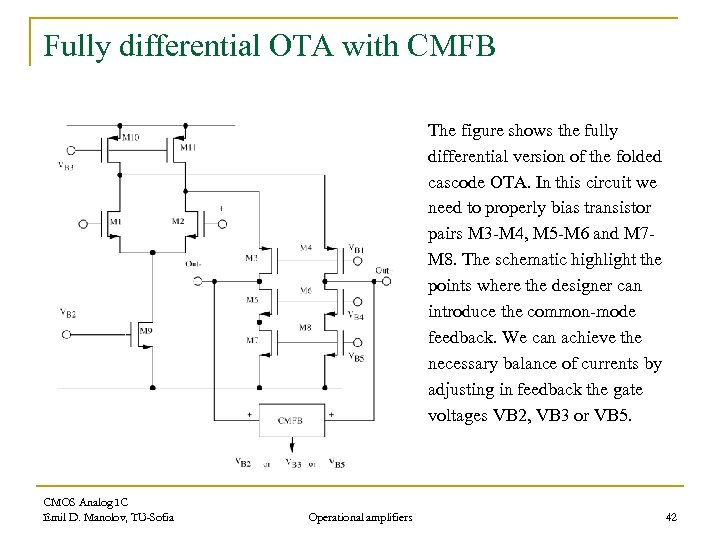Fully differential OTA with CMFB The figure shows the fully differential version of the folded cascode OTA. In this circuit we need to properly bias transistor pairs M 3 -M 4, M 5 -M 6 and M 7 M 8. The schematic highlight the points where the designer can introduce the common-mode feedback. We can achieve the necessary balance of currents by adjusting in feedback the gate voltages VB 2, VB 3 or VB 5. CMOS Analog IC Emil D. Manolov, TU-Sofia Operational amplifiers 42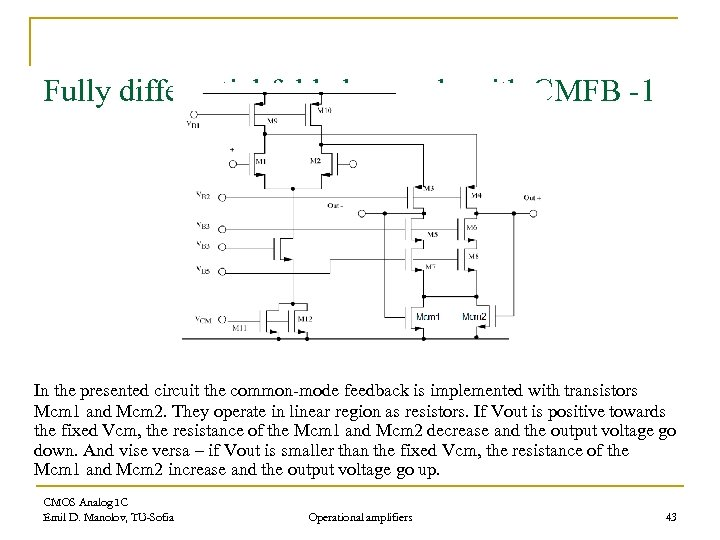Fully differential folded cascode with CMFB -1 In the presented circuit the common-mode feedback is implemented with transistors Mcm 1 and Mcm 2. They operate in linear region as resistors. If Vout is positive towards the fixed Vcm, the resistance of the Mcm 1 and Mcm 2 decrease and the output voltage go down. And vise versa – if Vout is smaller than the fixed Vcm, the resistance of the Mcm 1 and Mcm 2 increase and the output voltage go up. CMOS Analog IC Emil D. Manolov, TU-Sofia Operational amplifiers 43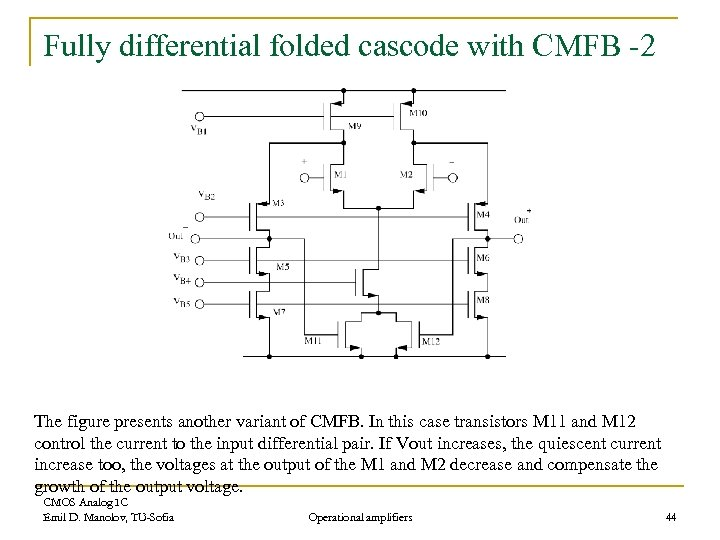Fully differential folded cascode with CMFB -2 The figure presents another variant of CMFB. In this case transistors M 11 and M 12 control the current to the input differential pair. If Vout increases, the quiescent current increase too, the voltages at the output of the M 1 and M 2 decrease and compensate the growth of the output voltage. CMOS Analog IC Emil D. Manolov, TU-Sofia Operational amplifiers 44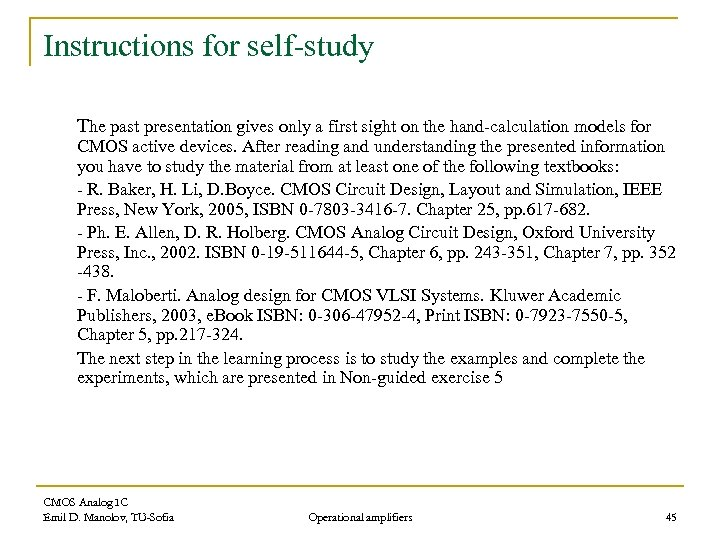Instructions for self-study The past presentation gives only a first sight on the hand-calculation models for CMOS active devices. After reading and understanding the presented information you have to study the material from at least one of the following textbooks: - R. Baker, H. Li, D. Boyce. CMOS Circuit Design, Layout and Simulation, IEEE Press, New York, 2005, ISBN 0 -7803 -3416 -7. Chapter 25, pp. 617 -682. - Ph. E. Allen, D. R. Holberg. CMOS Analog Circuit Design, Oxford University Press, Inc. , 2002. ISBN 0 -19 -511644 -5, Chapter 6, pp. 243 -351, Chapter 7, pp. 352 -438. - F. Maloberti. Analog design for CMOS VLSI Systems. Kluwer Academic Publishers, 2003, e. Book ISBN: 0 -306 -47952 -4, Print ISBN: 0 -7923 -7550 -5, Chapter 5, pp. 217 -324. The next step in the learning process is to study the examples and complete the experiments, which are presented in Non-guided exercise 5 CMOS Analog IC Emil D. Manolov, TU-Sofia Operational amplifiers 45